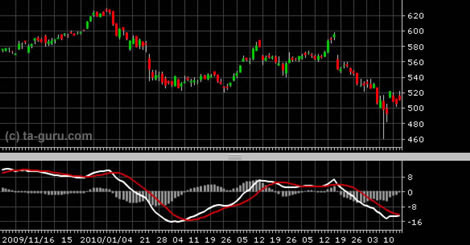Chart Pro online
User name (email):

 Recently used

 Forex

 Indices

# Moving Average Convergence Divergence (MACD)

Moving Average Convergence / Divergence (MACD) was created by Gerald Appel. This indicator combines oscillators and two exponential moving averages. It is calculated as the difference between the value of exponential moving averages (EMA) for a short period (e.g. 12) and for a longer period (e.g. 24). It includes also the line that is used for generating buying and selling signals. It is called the signal line and it is typically calculated for 9 samples.

Formulas for calculating the indicators are:

 MACD = EMA (CLOSE, 12) - EMA (CLOSE, 24) SIGNAL = SMA (MACD; 9)Moving average convergence divergence (MACD)

Interpretation

Intersections

When the MACD line crosses below the signal line that is a good time to sell. Also, if the MACD line crosses above the signal line it's time to buy.

Divergence

If price falls to new low, but MACD fails to reach new low, downward trend is losing its power and we could expect trend reversal in the near future. Similar goes for upward trend: if MACD fails to reach new high when price hits new high, upward trend is losing its power.

Dramatic growth

When the MACD line starts to grow significantly, it means that the price is overbought and soon will decline.

Intersection with zero line

Buying and selling signals can be generated by comparison of MACD line with the zero line. When MACD crosses above zero line it is a clear sign that the trend has changed from downward to upward trend. Opposite goes when MACD crosses below zero line: trend is changed from upward to downward.

Histogram

On MACD chart panel usually is drawn histogram. Histogram represents the difference between the value of MACD and signal line. When the histogram is negative and the value starts approaching to zero line, it means that the downward trend is losing strength. Similar is true for positive values approaching to zero line, they indicate that the upward trend loses its strength.

Example